# Class 10 Maths NCERT Solutions for Similar triangles EXERCISE 6.4

In this page we have Class 10 Maths NCERT Solutions for Similar triangles for EXERCISE 6.4 on pages 143 and 144. Hope you like them and do not forget to like , social_share and comment at the end of the page.
Formula Used in this exercise
(i) AA or AAA Similarity Criterion:
If all the angles or two angles are equal between two triangles, then Triangles are similar and corresponding sides are in proportion

(ii) SSS Similar Criterion:
If in two triangles, sides of one triangle is proportion to other triangle, then triangles are similar and corresponding angles will be equal between the triangles
(iii) SAS Similar Criterion:
If one angle is equal to one angle of another triangle and sides including those angles are proportional, the triangles are similar

## Triangles EXERCISE 6.4

Question 1.
Let ΔABC ~ ΔDEF and their areas be, respectively, 64 cm2and 121 cm2. If EF = 15.4 cm, find BC.
It is given that,
Area of ΔABC = 64 cm2
Area of ΔDEF =121 cm2
EF = 15.4 cm
and, ΔABC ~ ΔDEF
If two triangles are similar, ratio of their areas are equal to the square of the ratio of their corresponding sides
Therefore
$\frac {Area \Delta ABC}{Area \Delta DEF} = \frac {BC^2}{EF^2}$
$\frac {64}{121} = \frac {BC^2}{EF^2}$
$\frac {64}{121} = \frac {BC^2}{15.4^2}$?
Solving, we get
BC = 11.2 cm

Question 2.
Diagonals of a trapezium ABCD with AB || DC intersect each other at the point O. If AB = 2CD, find the ratio of the areas of triangles AOB and COD.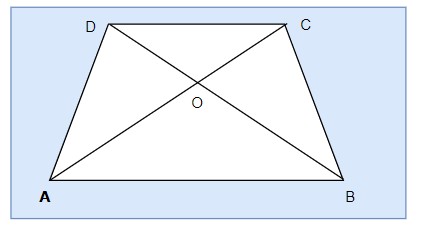ABCD is a trapezium with AB || DC. Diagonals AC and BD intersect each other at point O.
In ΔAOB and ΔCOD, we have
∠OBA= ∠ODC(Alternate angles)
∠OAB= ∠OCD(Alternate angles)
∠AOB= ∠COD(Vertically opposite angle)
By AAA similarity criterion
ΔAOB ~ ΔCOD
Now we know that if two triangles are similar then the ratio of their areas are equal to the square of the ratio of their corresponding sides
Therefore
$\frac {Area \Delta AOB}{Area \Delta COD} = \frac {AB^2}{CD^2}$
Now AB = 2CD
$\frac {Area \Delta AOB}{Area \Delta COD} = \frac {(2CD)^2}{CD^2}$
$\frac {Area \Delta AOB}{Area \Delta COD} = \frac {4}{1}$
Hence, the required ratio of the area of ΔAOB and ΔCOD = 4:1

Question 3.
In the below figure, ABC and DBC are two triangles on the same base BC. If AD intersects BC at O, show that area (ΔABC)/area (ΔDBC) = AO/DO.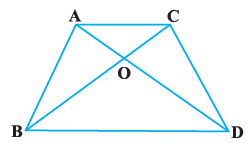Given: ABC and DBC are triangles on the same base BC. ADintersects BC at O.
Construction: Let us draw two perpendiculars AP and DQon line BC.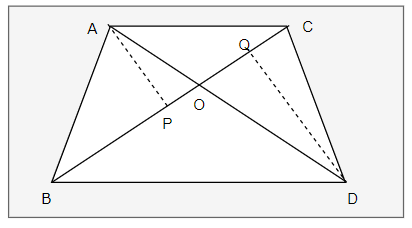Now
area of a triangle = 1/2 × Base × Height
Therefore
$\frac {area (\Delta ABC)}{area (\Delta DBC)} = \frac { 1/2 \times BC \times AP} {1/2 \times BC \times DQ}$
$\frac {area (\Delta ABC)}{area (\Delta DBC)} = \frac {AP}{DQ}$ -(1)
Now
In ΔAPO and ΔDQO,
∠APO = ∠DQO (Each equals to 90°)
∠AOP = ∠DOQ(Vertically opposite angles)
By AA similarity criterion
ΔAPO ~ ΔDMO
Now from Similarty equation
$\frac {AP}{DQ}= \frac {AO}{DO}$ -(2)
From equation (1) and (2)
area (ΔABC)/area (ΔDBC) = AO/DO

Question 4.
If the areas of two similar triangles are equal, prove that they are congruent.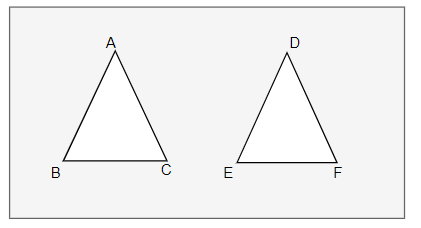Given: ΔABC and ΔDEF are similar and equal in area.
Now we know that if two triangles are similar then the ratio of their areas are equal to the square of the ratio of their corresponding sides
Therefore
$\frac {Area \Delta ABC}{Area \Delta DEF}= \frac {BC^2}{EF^2}$
Now as area are equal
$\frac {BC^2}{EF^2} =1$
BC = EF
Similarly, we can prove that
AB = DEand AC = DF
By SSS criterion of congruence
Therefore
ΔABC ≅ ΔDEF

Question 5.
D, E and F are respectively the mid-points of sides AB, BC and CA of ΔABC. Find the ratio of the area of ΔDEF and ΔABC.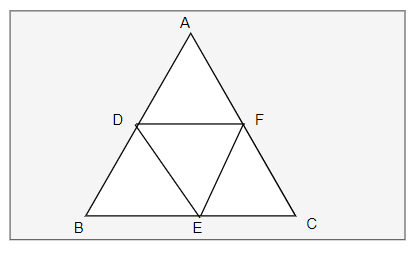Given: D, E and F are the mid-points of the sides AB, BC and CA respectively of the ΔABC.
So, by the mid-point theorem, we have
DE || AC and DE = 1/2 AC

In ΔBED and ΔBAC, we have
∠BED=∠BAC
∠BDE=∠BAC
By AA similarity criterion
ΔBED~ ΔBAC
Now we know that if two triangles are similar then the ratio of their areas are equal to the square of the ratio of their corresponding sides
Therefore
$\frac {Area \Delta BED}{Area \Delta BAC}= \frac {DE^2}{AC^2}= \frac {1}{4}$
or
$Area \; \Delta BED =\frac {1}{4} Area \; \Delta BAC$
Let $Area \; \Delta BAC =x$
$Area \; \Delta BED =\frac {1}{4} x$
Similarly, we can prove that
$Area \; \Delta ADF =\frac {1}{4} x$
$Area \; \Delta CEF =\frac {1}{4} x$
Now, area(ΔABC) = area(ΔBED)+ area(ΔDEF)+ area(ΔADF)+area(ΔCEF)
$x=\frac {1}{4} x +\frac {1}{4} x +\frac {1}{4} x + area(\Delta DEF)$
or
area(ΔDEF) = 1/4 area(ΔABC)
Hencearea(ΔDEF)/area(ΔABC) = 1/4

Question 6.
Prove that the ratio of the areas of two similar triangles is equal to the squareof the ratio of their corresponding medians.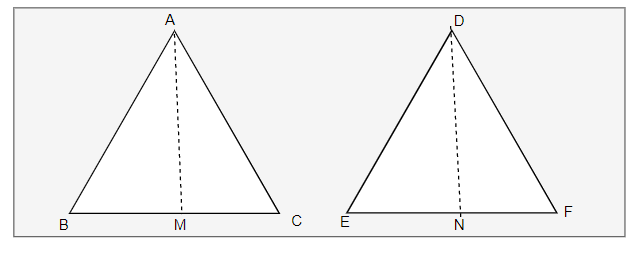Given: AM and DN are the medians of triangles ABC and DEF respectively and ΔABC ~ ΔDEF.
Now
Now we know that if two triangles are similar then the ratio of their areas are equal to the square of the ratio of their corresponding sides
area(ΔABC)/area(ΔDEF) = (AB2/DE2) .
Also from similarity theoreom
$\frac { AB}{DE} = \frac {BC}{EF} = \frac {CA}{FD}$
Now as $BM =\frac {1}{2} BM$ and $EF =\frac {1}{2} EN$
We can write as
$\frac { AB}{DE} = \frac {BM}{EN}$

In ΔABM and ΔDEN, we have
∠B = ∠E
$\frac { AB}{DE} = \frac {BM}{EN}$
By SAS similarity criterion
ΔABM ~ ΔDEN
Now from similarity
$\frac {AB}{DE} = \frac {AM}{DN}$ .

As the areas of two similar triangles are proportional to the squares of the corresponding sides.
area(ΔABC)/area(ΔDEF) = AB2/DE2= AM2/DN2

Question 7.
Prove that the area of an equilateral triangle described on one side of a square is equal to half the area of the equilateral triangle described on one of its diagonals.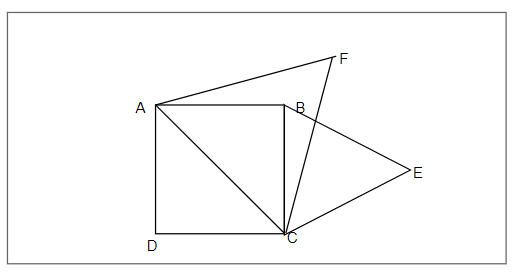ABCD is a square whose one diagonal is AC. ΔACF and ΔBCEare two equilateral triangles described on the diagonals AC and side BC of the square ABCD.
ΔACFand ΔBCEare both equilateral triangles (Given)
AAA similarity criterion
ΔACF~ ΔBCE
Therefore
area(ΔACF)/area(ΔBCE) = (AC2/BC2) = AC2/BC2
Now $AC = \sqrt {2} BC$
Therefore
area(ΔBCE) = (1/2) area(ΔACF)

Tick the correct answer and justify:

Question 8.
ABC and BDE are two equilateral triangles such that D is the mid-point of BC. Ratio of the area of triangles ABC and BDE is
(A) 2 : 1
(B) 1 : 2
(C) 4 : 1
(D) 1 : 4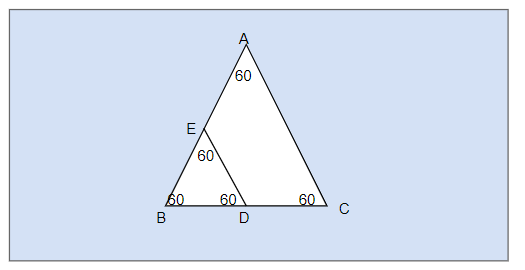ΔABC and ΔBDE are two equilateral triangle. D is the mid point of BC.
∴ $BD = DC = \frac {1}{2} BC$
Let each side of triangle is 2b.
As, ΔABC ~ ΔBDE (Both equilateral triangles)
∴ area(ΔABC)/area(ΔBDE) = AB2/BD2= (2b)2/(b)2= 4b2/b2= 4/1 = 4:1
Hence, the correct option is (C).

Question 9.
Sides of two similar triangles are in the ratio 4 : 9. Areas of these triangles are in the ratio
(A) 2 : 3
(B) 4 : 9
(C) 81 : 16
(D) 16 : 81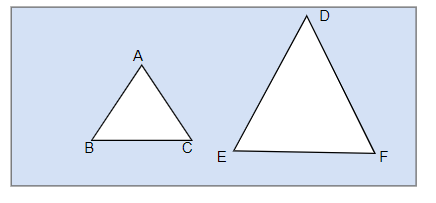Let ABC and DEF are two similarity triangles ΔABC ~ ΔDEF (Given)
and $\frac {AB}{DE} = \frac {AC}{DF} = \frac {BC}{EF} = \frac {4}{9}$ (Given)
No the ratio of the areas of these triangles will be equal to the square of the ratio of the corresponding sides ∴ area(ΔABC)/area(ΔDEF) = AB2/DE2
area(ΔABC)/area(ΔDEF) =(4/9)2= 16/81 = 16:81
Hence, the correct option is (D).

## Summary

1. NCERT Solutions for Class 10 Maths: Chapter 6 triangles Exercise 6.4 has been prepared by Expert with utmost care. If you find any mistake.Please do provide feedback on mail.You can download this as pdf
2. This chapter 6 has total 5 Exercise 6.1 ,6.2,6.3 ,6.4 and 6.5. This is the Fourth exercise in the chapter.You can explore previous exercise of this chapter by clicking the link belowGo back to Class 10 Main Page using below links

### Practice Question

Question 1 What is $1 - \sqrt {3}$ ?
A) Non terminating repeating
B) Non terminating non repeating
C) Terminating
D) None of the above
Question 2 The volume of the largest right circular cone that can be cut out from a cube of edge 4.2 cm is?
A) 19.4 cm3
B) 12 cm3
C) 78.6 cm3
D) 58.2 cm3
Question 3 The sum of the first three terms of an AP is 33. If the product of the first and the third term exceeds the second term by 29, the AP is ?
A) 2 ,21,11
B) 1,10,19
C) -1 ,8,17
D) 2 ,11,20